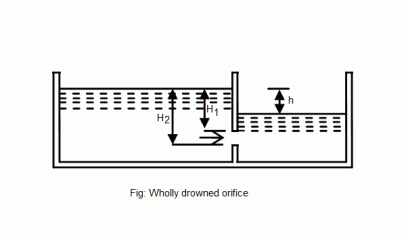•http://facebook.com/
•https://www.google.com/accounts/o8/id
•https://me.yahoo.com

# Submerged Orifice

Discharge through a submerged orifice

## Discharge Through A Wholly Drowned Orifice

When the outlet side of an orifice is beneath the surface of liquid it is known as a wholly submerged orifice as shown in fig.1. In such orifices, the coefficient of contraction is equal to one.Consider a wholly drowned orifice discharging water as shown in fig.1.

Let,
• = Height of water (on the upstream side) above the top of the orifice
• = Height of water (on the upstream side) above the bottom of the orifice
• = Difference between the two water levels on either side of the orifice
• = Coefficient of discharge
• = Coefficient of velocity
• = Coefficient of contraction

Area of orifice =

We know that the theoretical velocity of water through the strip =

Actual velocity of water =

From the relation of hydraulic coefficients we know that,

Since coefficient of contraction is 1 in this case, therefore

Actual velocity of water =

Now the discharge through the orifice,

= Area of orifice Actual velocity

If depth of the drowned orifice (d) is given instead of and , then in such cases the discharge through the wholly drowned orifice is:

Example:
[metric]
##### Example - Discharge through a wholly drowned orifice
Problem
A drowned orifice 1.5m wide and 0.5m deep is provided in one side of a tank. Find the discharge in liters/s through the orifice, if the difference of water levels on both the sides of the orifice be 4m. Take = 0.64.
Workings
Given,
• = 1.5m
• = 0.5m
• = 4m
• = 0.64

Solution
Discharge = 4250 liters/s